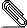[Wien] “Empty” states are populated during SCF

Hi List,

I have a big calculation running with MSR1a (one of those oxide surfaces
I asked about earlier).  After a while, it stopped due to touching
spheres, so I used clminter with `setrmt -r 3' and restarted.  After 130
more iterations, the calculation stops with a QTL-B error

QTL-B VALUE .EQ.    9.42627   in Band of energy   -0.21251   ATOM=
129   L=  3
:WARN : QTL-B value eq.  29.45 in Band of energy  -0.35946  ATOM=  134
L=  3

Now, atom 134 is a La and its f-states should be *empty*.  And so they
were for most of the calculation, but ~30 iterations after clminter,
they start to be full (they pretty much jump from 0 to 7/spin, here is a
plot: <http://i.imgur.com/DZavhiq.png>; the scale goes to 7).

This actually runs (with some oscillations) for ~100 iterations, then
the QTL-B error happens.

What could be the cause of this?

The R-MT for La is 2.28, before the clminter it was 2.35.  ‘scf1’ section:

ATOMIC SPHERE DEPENDENT PARAMETERS FOR ATOM  La lo
:e__0134: OVERALL ENERGY PARAMETER IS    0.2434
OVERALL BASIS SET ON ATOM IS LAPW
:E0_0134: E( 0)=   -2.9884   E(BOTTOM)=   -3.787   E(TOP)=   -2.190  4
5   177
APW+lo
:E0_0134: E( 0)=    0.6434
LOCAL ORBITAL
:E1_0134: E( 1)=   -1.5836   E(BOTTOM)=   -2.710   E(TOP)=   -0.457  3
4   218
APW+lo
:E1_0134: E( 1)=    0.6434
LOCAL ORBITAL
:E2_0134: E( 2)=    0.2434   E(BOTTOM)=   -0.980   E(TOP)= -200.000  2
-1   175
APW+lo

Thanks,

Elias

--
Elias Assmann
Institute of Theoretical and Computational Physics
TU Graz                   ⟨https://itp.tugraz.at/⟩signature.asc
Description: OpenPGP digital signature

_______________________________________________
Wien mailing list
Wien@zeus.theochem.tuwien.ac.at
http://zeus.theochem.tuwien.ac.at/mailman/listinfo/wien
SEARCH the MAILING-LIST at:
http://www.mail-archive.com/wien@zeus.theochem.tuwien.ac.at/index.html